## Maximum Likelihood Phylogeny

To generate a maximum likelihood based phylogenetic tree:

Toolbox | Classical Sequence Analysis () | Alignments and Trees (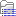)| Maximum Likelihood Phylogeny (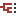)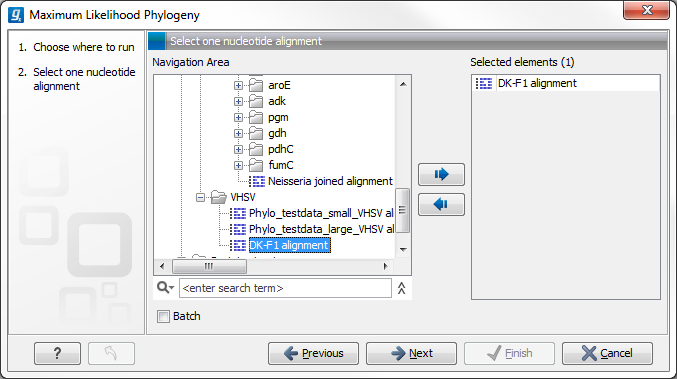Figure 20.7: Select the alingment for tree construction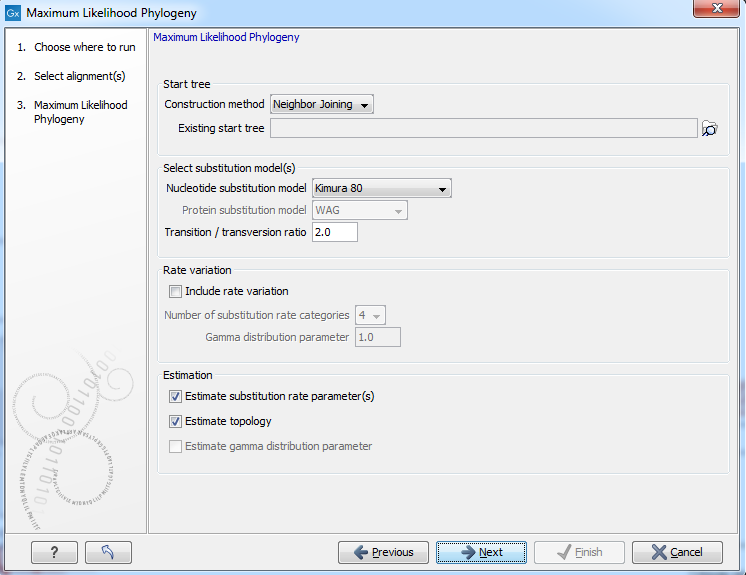Figure 20.8: Adjusting parameters for maximum likelihood phylogeny

The following parameters can be set for the maximum likelihood based phylogenetic tree (see figure 20.8):

• Starting tree
• Construction method Specify the tree construction method which should be used to create the initial tree. There are two possibilities:
• Neighbor Joining
• UPGMA

• Exiting start tree Alternatively, an existing tree can be used as starting tree for the tree reconstruction. Click on the folder icon to the right of the text field to use the browser function to identify the desired starting tree.

• Select substitution model
• Nucleotice substitution model CLC Genomics Workbench allows maximum likelihood tree estimation to be performed under the assumption of one of five nucleotide substitution models:

All models are time-reversible. In the Kimura 80 and HKY models, the user may set a transtion/transversion ratio value, which will be used as starting value for optimization or as a fixed value, depending on the level of estimation chosen by the user. For further details, see 20.2.5.

The Bishop-Friday substitution model is similar to the Jukes-Cantor model for nucleotide sequences, i.e. it assumes equal amino acid frequencies and substitution rates. This is an unrealistic assumption and we therefore recommend using one of the remaining three models. The Dayhoff, JTT and WAG substitution models are all based on large scale experiments where amino acid frequencies and substitution rates have been estimated by aligning thousands of protein sequences. For these models, the maximum likelihood tool does not estimate parameters, but simply uses those determined from these experiments.

• Rate variation

To enable variable substitution rates among individual nucleotide sites in the alignment, select the include rate variation box. When selected, the discrete gamma model of Yang [Yang, 1994b] is used to model rate variation among sites. The number of categories used in the discretization of the gamma distribution as well as the gamma distribution parameter may be adjusted by the user (as the gamma distribution is restricted to have mean 1, there is only one parameter in the distribution).

• Estimation

Estimation is done according to the maximum likelihood principle, that is, a search is performed for the values of the free parameters in the model assumed that results in the highest likelihood of the observed alignment [Felsenstein, 1981]. By ticking the estimate substitution rate parameters box, maximum likelihood values of the free parameters in the rate matrix describing the assumed substitution model are found. If the Estimate topology box is selected, a search in the space of tree topologies for that which best explains the alignment is performed. If left un-ticked, the starting topology is kept fixed at that of the starting tree.

The Estimate Gamma distribution parameter is active if rate variation has been included in the model and in this case allows estimation of the Gamma distribution parameter to be switched on or off. If the box is left un-ticked, the value is fixed at that given in the Rate variation part. In the absence of rate variation estimation of substitution parameters and branch lengths are carried out according to the expectation maximization algorithm[Dempster et al., 1977]. With rate variation the maximization algorithm is performed. The topology space is searched according to the PHYML method [Guindon and Gascuel, 2003], allowing efficient search and estimation of large phylogenies. Branch lengths are given in terms of expected numbers of substitutions per nucleotide site.

In the next step of the wizard it is possible to perform bootstrapping (figure 20.9).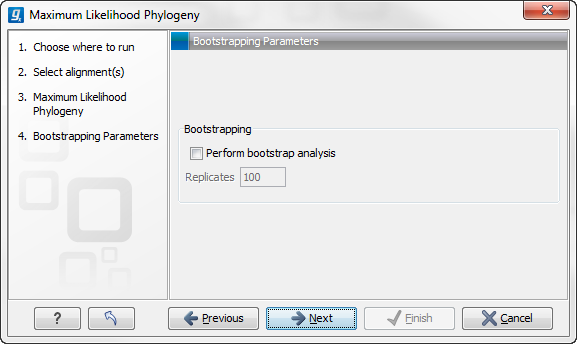Figure 20.9: Adjusting parameters for ML phylogeny

• Bootstrapping
• Perform bootstrap analysis. To evaluate the reliability of the inferred trees, CLC Genomics Workbench allows the option of doing a bootstrap analysis (see Bootstrap tests). A bootstrap value will be attached to each node, and this value is a measure of the confidence in the subtree rooted at the node. The number of replicates in the bootstrap analysis can be adjusted in the wizard by specifying the number of times to resample the data. The default value is 100 resamples. The bootstrap value assigned to a node in the output tree is the percentage (0-100) of the bootstrap resamples which resulted in a tree containing the same subtree as that rooted at the node.